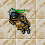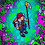## Wednesday, December 21, 2022

1.2.Tamarizz - Solidera

The arrow from the plus sign (+) to the other side of the equation turning it into a subtraction (-), and the II from the left turning it into a III

X - VII = III

3.X-Vlll=lll
Ajmeh - Solidera

4.X-VII=III

Hefrus

5.6.X + VII = II
X - VII = III
10 - 7 = 3

Nick: The Heringer

7.X-VII=III

8.9.10.IX-VII=II

EXLIPHERX - HAVERA

11.12.IX - VII = II

Inkkz

13.Meregirl
X - VIII = II

14.15.Presckot
X-VII=III

16.Lyh

X-VII=III

17.X - VII = III
SHIZURIE

18.X-VII=III
Xavlar

19.20.21.X-VII=III

sir coor

22.Name Char: Hinata Hyuga Byakugan

Respuesta: X - VIII = II

23.Mover la flecha vertical del signo de ( + ) del lado derecho para formar el numero tres; entonces seria x(10) - (v(5)II(2)) = 3 ::::::::::::::::::::::::::::::::::::::::::::::::::::::
Move the vertical arrow that makes the ( + ) sign to the right side to make the numer III (3); then it will be x(10) - (v(5)II(2)) = 3

24.Move the vertical arrow from the plus to make it minus and then we add it to VII to make it VIII so it ends as:
X - VIII = II

25.X-VII=III

Righo

26.Pan Ceta

X-Vll=lll

27.x - vii = iii

10-7= 10

Lervan

28.Psykeeh

X - VII = III

29.10 - 7 = 3
Char: Duli Leos

30.X - VII = III
Bea Charlover Aca

31.I think it should be:
X - VIII = II so just move an arrow from + to make it - and add it to VII to make it VIII
-Sapo Dungeon

32.X - VIII = II so just move an arrow from + to make it - and add it to VII to make it VIII
-Bara de pan

33.Respuesta: IX - VII = II

34.X - VII = III

Babygirrl

35.X - VII = III
Green Khepri

36.X - VII = III

Soma deux

37.Name Char: Kathryna

Respuesta: X - VIII = II

38.x - viii = ii
(x - 8 = 2 )

Ramona Toledo

39.R= IX - VII = II
X - VIII = II
Samuray de carro :)

40.R= IX - VII = II
X - VIII = II
Samuray de carro :)

41.The equation is X+VII=II (10+7=2)
to make the equation correct it should be:
IX-VII=II (9-7=2)

Character Name: Dyaniixz

42.The equation is X+VII=II (10+7=2)
to make the equation correct it should be:
IX-VII=II (9-7=2)

Character Name: Dyaniixz

43.correct equation: ix - vii = ii
character name: pablo reformed

44.correct equation: ix - vii = ii
character name: pablo reformed

45.Hi
I moved an arrow to make this figure IX - VII = II
Cinderella Sea

46.47.Kriizs
R: X - VII = III

48.Character name:Glexzs
World: Havera

A/ X - VII = III

49.X-VIII=II

50.X - VIII = II
X - VII = III

Amy Meow

51.Vertical arrow of the + moves to the end with II so it becomes - and III. X - VII = III.

-Essiee

52.X - VIII = II

Character: Mia Moonshine

53.I will go with X - VII = III

// Rand Silverfang